# Math Practice

Sharpen your math skills with these lessons and practice problems by Juni Math Instructors. Guide your learning with our explanations, tutorial videos, and solution walkthroughs.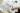## What Is Vertex Form? Unlocking the Elements of Quadratic Functions!

See how to use the vertex form of quadratic expressions and better understand quadratic functions and parabolas. More about the vertex form here!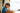## Understanding Quadrants for Math and in Graphing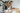## Using Multiples of 8 To Know What Least Common Multiple Means

The least common multiple tells us the smallest multiple shared between two or more numbers. This opens a wide range of applications, learn more here!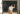## Why Is the Square Root of 25 Equal to 5? Time To Understand Principal Square Roots!

Principal square roots refer to the positive square root of any given positive number. The principal square root of 25 is equal to 5. Learn why here!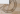## Identifying and Understanding Consecutive Interior Angles

Consecutive interior angles are angles found within the inner region of parallel lines on the same side of the transversal line. Learn more here!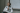## Exploring the Concept of Vertical Angles

Vertical angles are angles facing opposite each other in an intersecting line. They are always equal. Explore its concept and application here!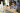## Understanding Ratios and Proportions Plus Proportional Relationships

Ratios are used to compare two or more companies. Proportions highlight the relationship of quantities using ratios. Learn more about ratios here!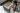## Knowing How To Find the Area and Perimeter of a Square

The area of a square is equal to the squares of its sides while its perimeter is equal to four times its side length. Master these two formulas here!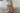## Understanding the Surface Area of a Cube and Its Applications

The surface area of a cube is equal to six times the square of its edge length. Learn how to find the surface area here and master its applications!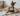## Mastering the Area of Triangles, Its Formula, and Applications

The area of a triangle is equal to half the product of its base and height. Check out this article to master the other ways to find its area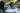## Mastering the Three Trigonometric Ratios: Sin Cos Tan

Sin cos tan are the trigonometric ratios that relate the sides and angles of a right triangle. Master sin cos tan here and learn how to apply them!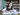## Understanding the Properties of Alternate Exterior Angles

Alternate exterior angles are formed when a transversal line cuts through parallel lines. Each pair of alternate exterior angles share equal measures.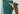## 3 Easy Methods for Converting Fractions and Decimals

Learn how to convert fractions and decimals using basic conversion, equivalent fractions, and long division in this simple math guide.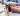## Associative Property of Addition: A Simple Tool for Growing Math Skills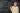## Factoring Higher Degree Polynomials

How, then, can we solve polynomials of higher degrees? By factoring! Here's a guide on how to factor.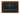## Coordinate Planes: Practicing Segments

Practice coordinate planes and segments with these drills and tutorial videos by Juni instructor Genesis.